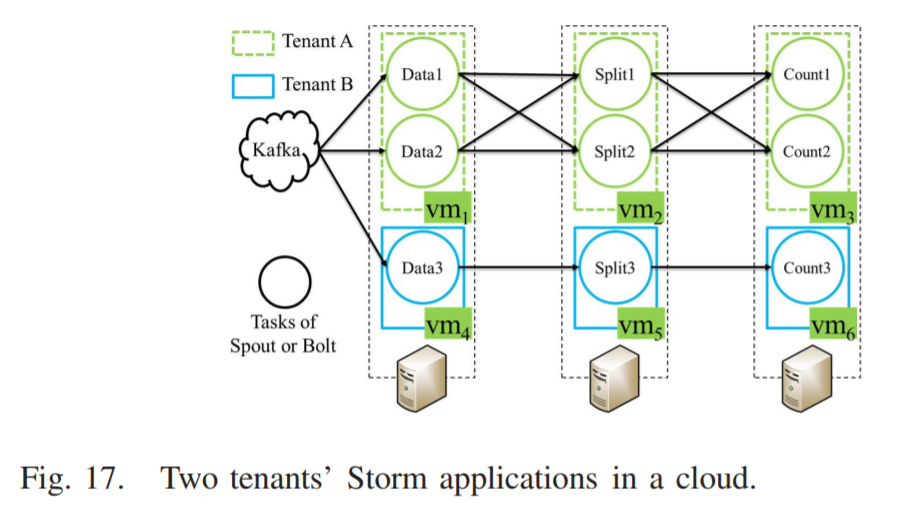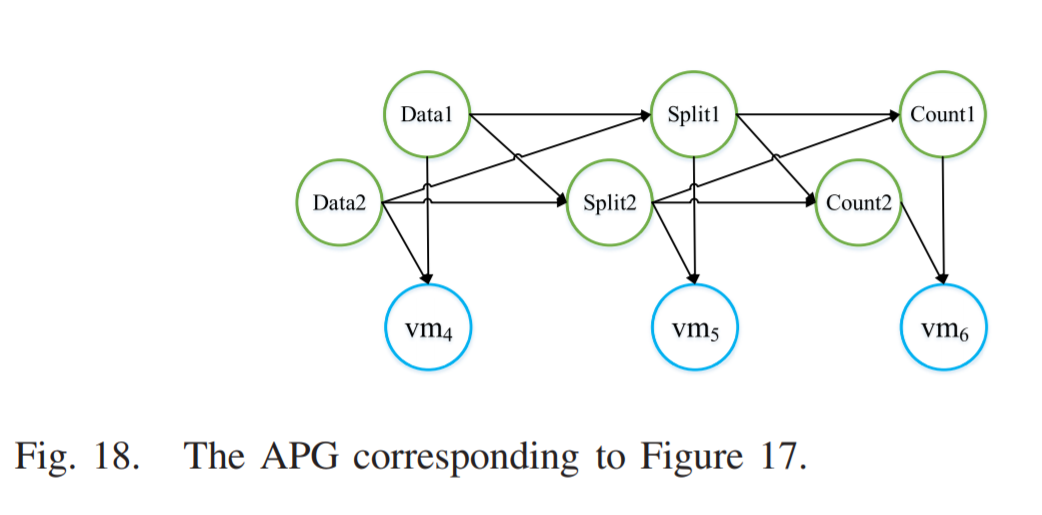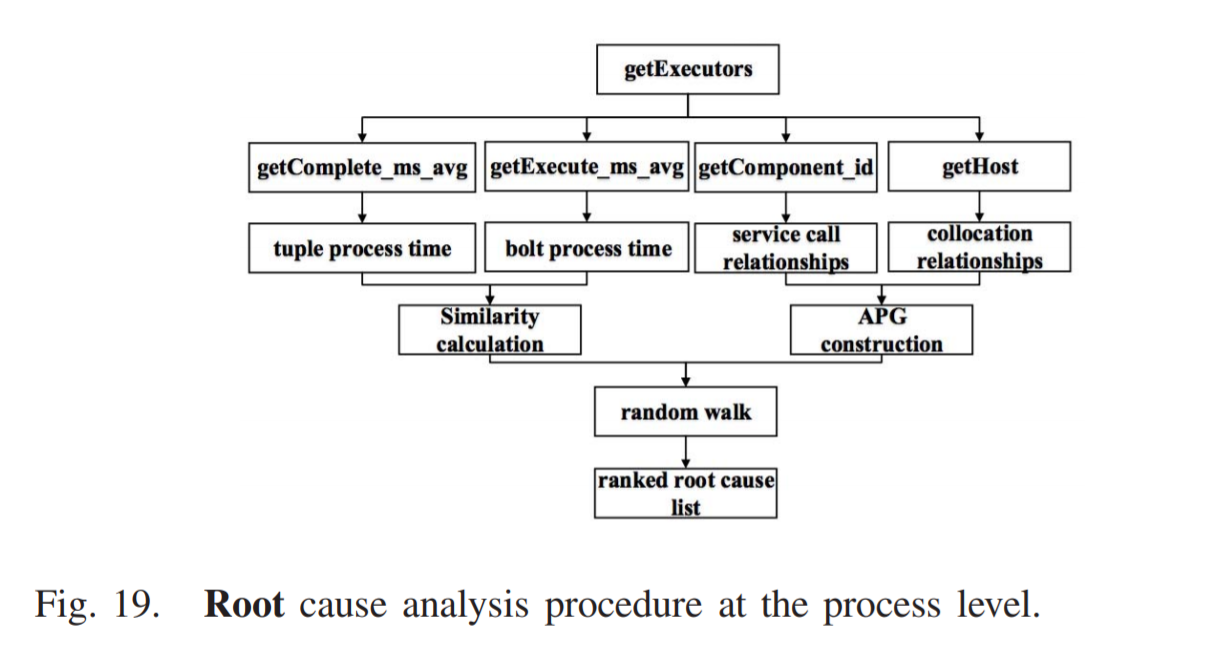# 简介

• 数据收集：对租户是非侵入性的，用以捕获多层服务组件的依赖关系并收集必要的服务度量数据
• 根本原因定位：找出可能引发异常的根本原因列表，以及每个可能的根本原因对应的概率
• 相似性评分的指标：基于服务的指标数据和底层基础设施的资源利用率进行计算
• 随机游走算法：模拟多层服务中异常传播的影响

# 相关工作

Pivot Tracing、伪异常聚类算法…… 云环境下应用程序的性能异常诊断。

• 利用特定于应用程序的知识或明确声明不同组件事件之间的因果关系，其缺点是用户必须获取和修改目标应用程序或中间件的源代码，而且不能用于黑箱服务；
• vPath ，可以在虚拟机外进行请求跟踪，但是 vPath 是在基于 XEN 的虚拟化环境中设计的，无法移植到基于 KVM 的虚拟化环境中；
• 在不了解源代码的情况下，通过内核检测或虚拟机内部的流量监控来推断因果路径
• 请求跟踪由 PreciseTracer 产生的因果路径图推断

# 异常传播类型和系统架构

## 异常传播类型

• 外部因素：虚拟机之间的资源争夺，异常可能从其他租户的虚拟机传播到该服务的一个虚拟机
• 内部因素：软件 bug，异常可能沿着服务调用的路径在多层服务的组件之间传播

• RUBiS 是一个为学术研究而开发的电子商务基准，可以根据研究人员设置的参数自动创建用户（10w）和商品（10w），初始化模拟一个在线购物网站；RUBiS 还提供了一个可模拟发送请求的用户行为的客户端，能够收集用户请求响应时间的统计结果。

• 租户 A 的 LVS 虚拟机根据用户请求的内容，将请求定向到两个 Apache 虚拟机中的一个（$vm_2$ 和 $vm_4$），即 $vm_1$ 实现了基于任务的负载平衡

• 负载平衡策略：使用 SearchItemsByRegion 函数和 ViewUserInfo 函数（$R_1$ 表示）的请求由 $vm_2$ 服务，使用 SearchItemsByCategory 函数和 ViewItem 函数的请求（$R_2$ 表示）由 $vm_4$ 服务

• 2 条调用路径

• $P_1$：$vm_1 \rightarrow vm_2 \rightarrow vm_3$

• $P_2$：$vm_1 \rightarrow vm_4 \rightarrow vm_5$

• 租户 B 只有 1 条调用路径 $P$ ：$vm_6 \rightarrow vm_7 \rightarrow vm_8$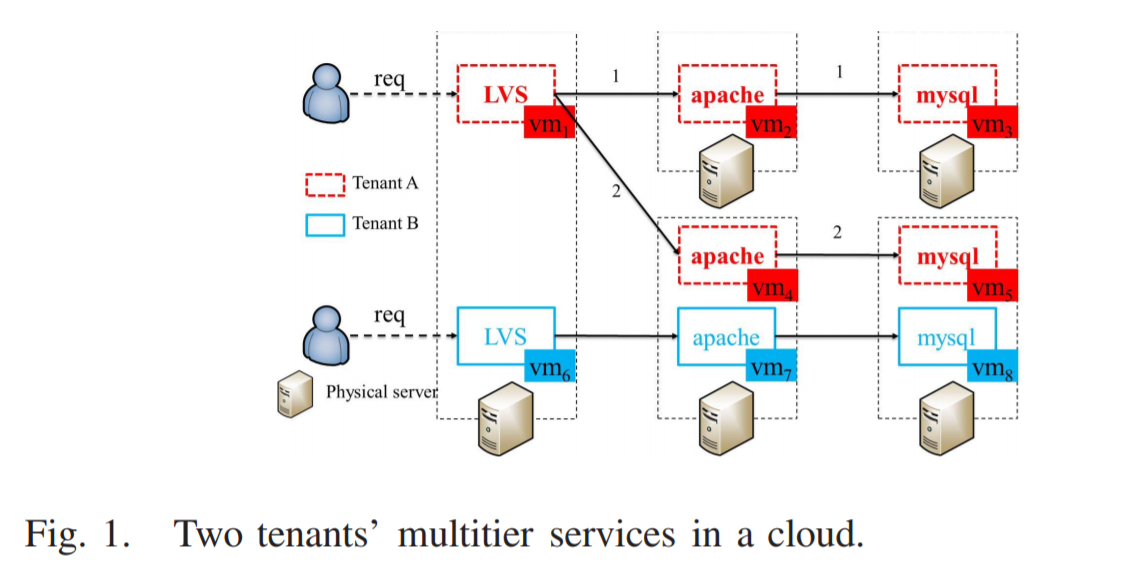• $vm_5$ 和 $vm_8$ 共享物理主机的 CPU 资源

• 2 种异常传播类型：$vm_8 \rightarrow vm_5$、$vm_5 \rightarrow vm_4 \rightarrow vm_1$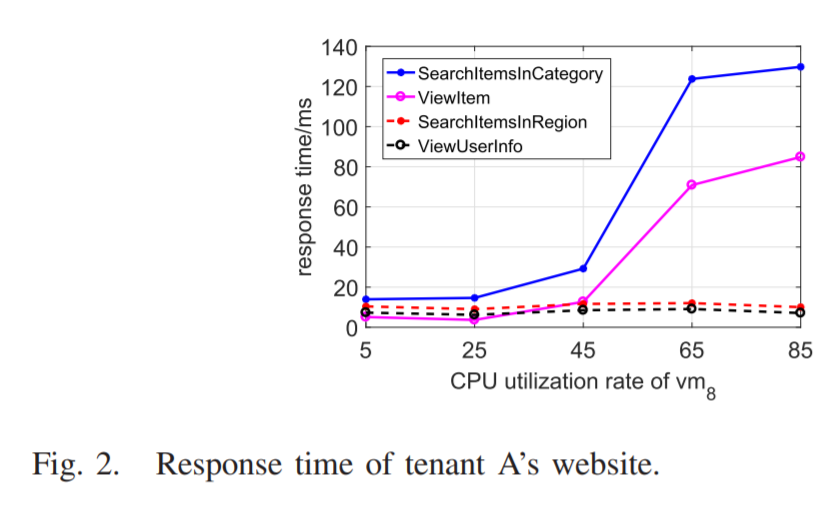## 系统架构

• 在线请求跟踪
• 度量数据收集

• 构建异常传播图（VCG）
• 构建虚拟机通信图（APG）
• 相似性计算
• 随机游走算法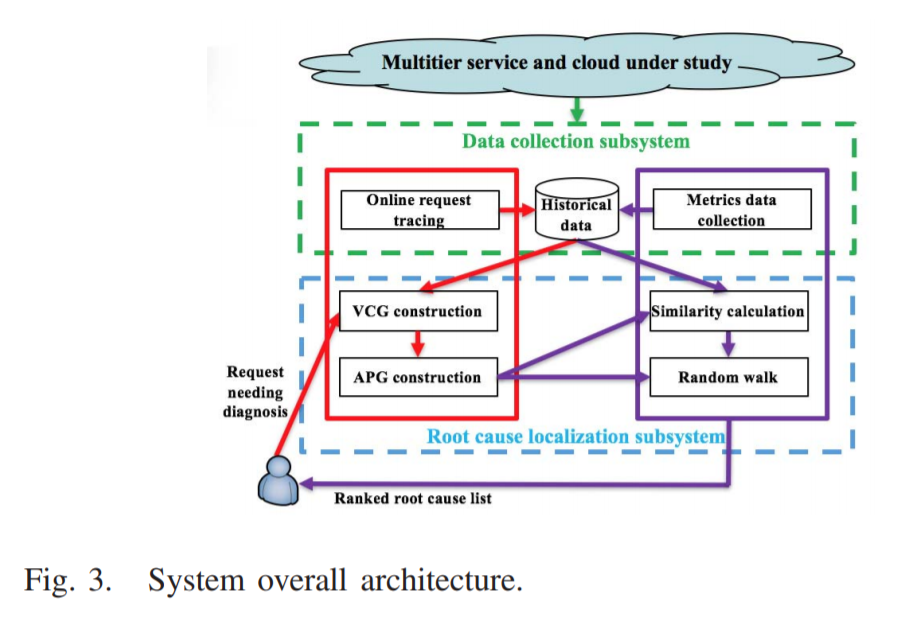• 找出所有可能导致异常 $a$ 的原因，并构建异常传播图（Anomaly Propagation Graph, APG）$G^{APG}_{a}$。

• 确定 $G^{APG}_{a}$ 中每个元素作为异常 $a$ 的根本原因的概率，并找出最可能的根本原因。

Step 1

Step 2

# 异常传播图（Anomaly Propagation Graph, APG）

$vm_i \rightarrow vm_j$ 表示 $vm_i$ 依赖 $vm_j$：事实上，这些依赖边是异常传播的反向方向

• 服务调用边：$vm_i$ 在处理请求时调用 $vm_j$

• 并置依赖边：$vm_i$ 和 $vm_j$ 位于同一个物理服务器中，而且 $vm_i$ 是多层服务的一个服务组件

## 多层服务请求跟踪

• BEGIN 活动标志着服务新请求的开始，END 活动标志着服务请求的结束

PreciseTracer 记录一个发送消息的活动为 $S^i_{i,j}$ ，表示进程 $i$ 向进程 $j$ 发送消息，记录一个接收消息的活动为 $R_j^{i,j}$，表示进程 $j$ 从进程 $i$ 接收一条消息。下图包含简单的活动序列 $\{R^1_{c,1},S^1_{1,2},R^2_{1,2},S^2_{2,3},R^3_{2,3},S^3_{3,x}\}$ ，根据这个图可以计算每个组件（虚拟机）在服务单个请求时的服务时间。对于下图中的请求，机器 B 的服务时间是 $t(S^2_{2,3})-t(R^2_{1,2})$ ，其中 $t(·)$ 为对应活动的时间戳，此外，定义 $h(·)$ 作为运行相应活动的虚拟机的主机名。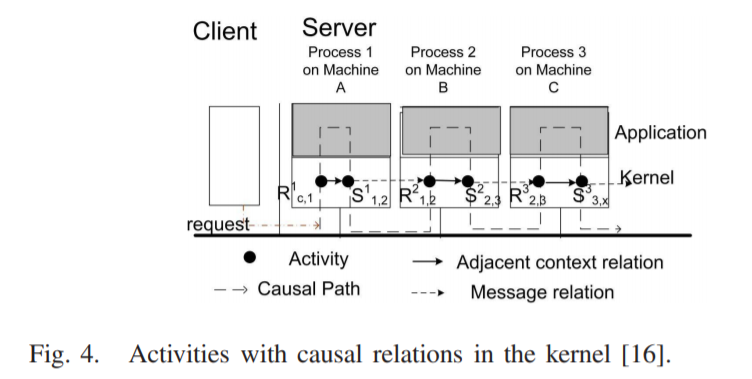## 构造 VCG

• 第一次调用：请求 $S^1_{1,2}$、$R^2_{1,2}$；响应 $S^2_{2,1}$、$R^1_{2,1}$

• 第二次调用：请求 $S^{1’}_{1,2}$、$R^{2’}_{1,2}$；响应 $S^{2’}_{2,1}$、$R^{1’}_{2,1}$

• 服务 2 调用 $vm_5$ 中的服务 3 两次，然后再返回给服务 1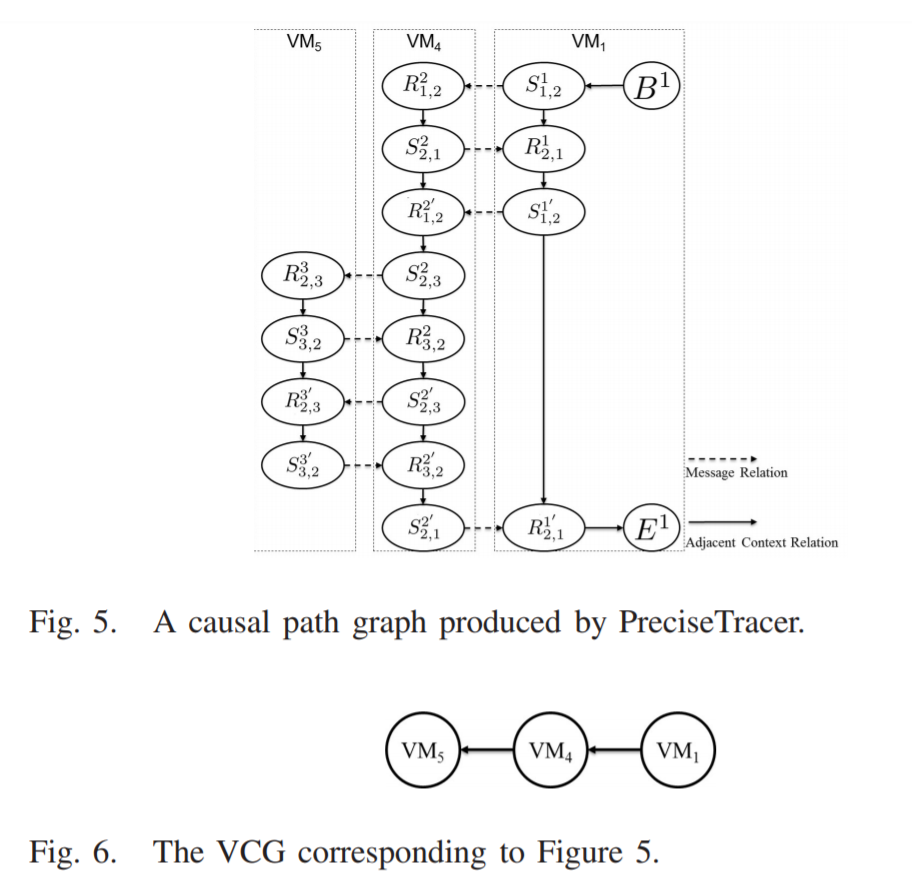• 根据每个活动的主机名，将因果路径图中的活动划分为不同的集合，活动集合 $\S{v}$ 包含所有与 $vm_v$ 相关的活动

• 对于每个虚拟机 $vm_v$，按照时间戳对 $\S{v}$ 中的活动进行排序

• 对于每个虚拟机 $vm_v$，尝试在区分服务调用的请求者和响应者的基础上确定其相关边的方向

• 对于 SEND 活动，找到对应的 RECEIVE 活动

• 找不到满足 $h(S^j_{j,i})=h(R^j_{j,i})$ 和 $t(S^j_{j,i})<t(R^j_{i,j})$ 的 SEND 活动 $S^j_{j,i}$ ，添加 $h(S^j_{j,i})\rightarrow h(R^j_{j,i})$

## 构造 APG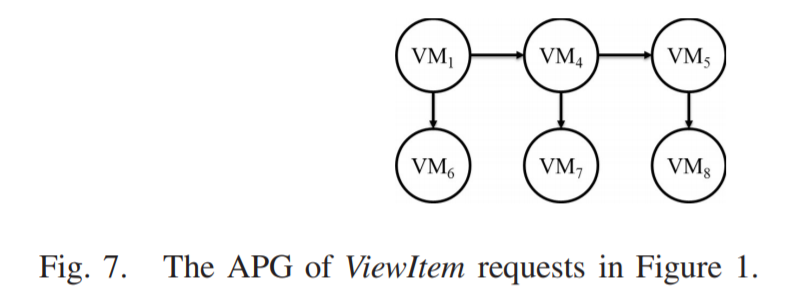# 根本原因定位

## 度量数据收集

1. 服务时间：虚拟机 $vm_i$ 对请求 $r$ 的服务时间表示为 $\eta_i = \sum_{x}(t(S^i_{i,x})-t(R^i_{x,i})) - \sum_{y}(t(R^i_{y,i})-t(S^i_{i,y}))$ ，$x$ 表示向虚拟机 $vm_i$ 发起请求的虚拟机，$y$ 表示接收虚拟机 $vm_i$ 请求的虚拟机；左式表示虚拟机 $vm_i$ 处理所有请求的时间和，包括等待响应时间，因此减去右式的等待间隔。

2. 资源消耗：使用 Ganglia 分布式监控系统收集资源利用率，默认收集物理机的度量数据，使用插件 sFlow 收集虚拟机的度量数据，包括 CPU 和内存消耗、I/O 和网络吞吐量。

## 相似度计算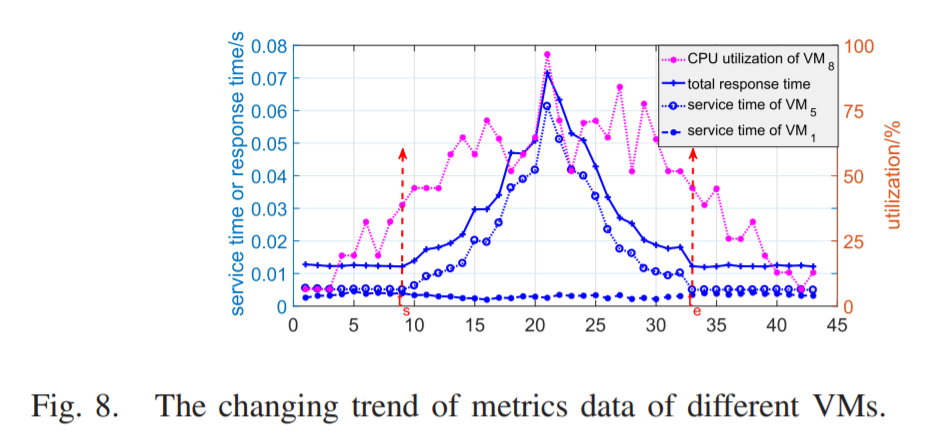$$\Re(i,M,\mathbb{R}(r),t_s,t_e) = \frac{Cov(\mathbb{M}^{t_e}_{t_s}(M,i),\mathbb{T}^{t_e}_{t_s}(\mathbb{R}(r)))}{\sigma_{\mathbb{M}^{t_e}_{t_s}(M,i)}\sigma_{\mathbb{T}^{t_e}_{t_s}(\mathbb{R}(r))}}$$

• $\mathbb{M}^{t_e}_{t_s}(M,i)$ 表示虚拟机 $vm_i$ 的度量数据

• $\mathbb{T}^{t_e}_{t_s}(\mathbb{R}(r))$ 表示相关请求的响应时间

$$\mathbb{S}(vm_i,\mathbb{R}(r))=\left\{ \begin{array}{rcl} \Re(i,M,\mathbb{R}(r),t_s,t_e), & \text{if}\ vm_i\in{VCG}\\ \max{\{\Re(i,M,\mathbb{R}(r),t_s,t_e)|v\in\Upsilon}\}, & \text{otherwise}\\ \end{array} \right.$$

• $\Upsilon$ 表示竞争的资源类型，例如 CPU、内存、I/O、网络
1. 在 $vm_i$ 处理请求 $\mathbb{R}(r)$ 时，收集 $vm_i$ 的服务时间 $\eta_i$ 的历史数据

给定一个因果路径图，根据拓扑排序算法，求得活动序列 $\S$，然后使用活动 $A$ 的属性元组（活动类型，程序名）来表示 $\S$ 中的活动 $A$ 。以图 5 为例，可以得到因果路径图序列为
$\{(B,P_1), (S, P_1), (R, P_2), (S, P_2), (R, P_1), (S, P_1), (R, P_2), (S, P_2), (R, P_3), (S, P_3), (R, P_2), (S, P_2), (R, P_3), (S, P_3), (R, P_2), (S, P_2), (R, P_1), (E,P_1)\}$ $B$、$E$、$S$、$R$ 表示四种活动，$P_i$ 表示进程 $i$ 的名称。最后，只需要找出与 $r$ 因果路径图具有相同序列的因果路径图。

2. 确定时间点 $t_s$ 和 $t_e$

$$t_s = \min{\{t|\frac{\tau(r_t)}{E(\mathbb{T}^t_{t-w}(\mathbb{R}(r)))}\}>\delta}$$

• $r_t$ 表示请求，$t$ 为时间戳，$w$ 为时间窗口长度

• $t_s$ 表示最早时间点 $t$，在 $t$ 时刻发出的请求响应时间与前一个时间窗口发出的请求的平均响应时间之比大于阈值 $\delta$ （例如，1.2）

## 随机游走算法

• 前向边

• 后向边：$\rho$ 由管理员设置，范围在 0~1 之间

• 重边

$$Q_{ij}=\left\{ \begin{array}{rcl} \mathbb{S}(vm_j,\mathbb{R}(r)), & \text{if}\ e_ij\in{E}\\ \rho\mathbb{S}(vm_j,\mathbb{R}(r)), & \text{if}\ e_{ji}\in{E},e_{ij}\notin{E}\\ \max{(0,\mathbb{S}(vm_i,\mathbb{R}(r))-\max_{k:e_j,k\in{E}}\mathbb{S}(vm_k,\mathbb{R}(r)))}, & \text{if}\ j=i\\ \end{array} \right.$$

# 实验评估

## 实验环境

• OpenStack：搭建云环境，提供虚拟机服务等。

• PreciserTracer：在每台虚拟机上部署代理 TCP_Tracer ，然后把不同虚拟机的活动日志关联到因果路径图中。从因果路径图中计算关于每个虚拟机服务时间的度量数据，并将结果存储在数据库中。

• Ganglia：部署在每个物理服务器上，收集每个虚拟机的资源利用率。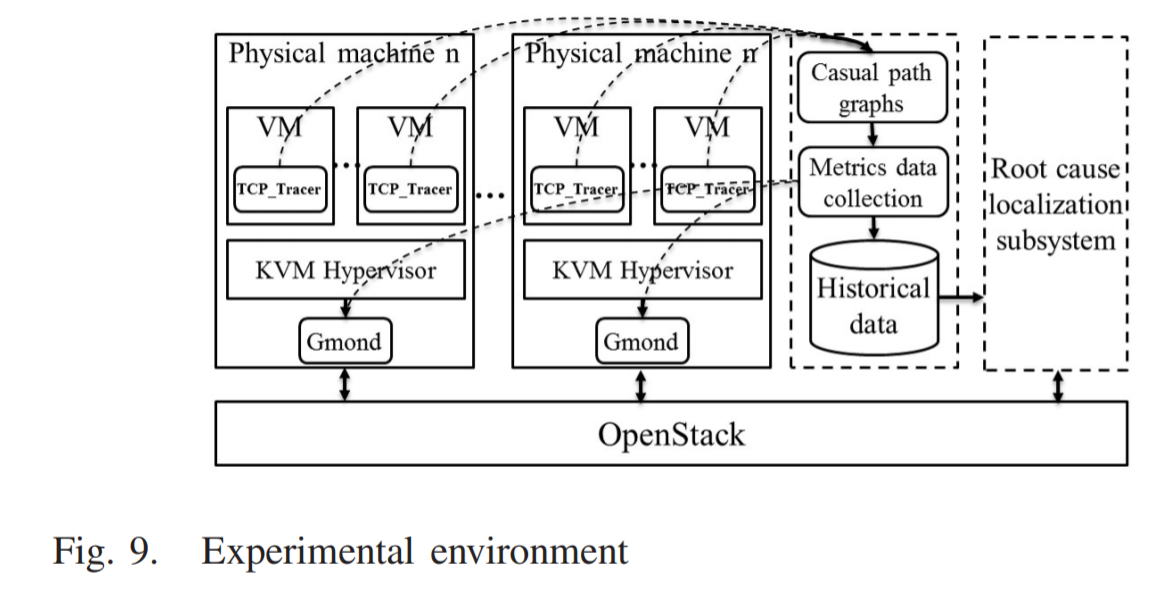## 性能评估

• 随机选择（Random Selection, RS）：模拟随机检查虚拟机
• 突变（Sudden Change, SC）：比较当前时间窗口和以前时间窗口的度量比率
• 基于距离的排名（Distance Based Rank, DBR）：每个组件 $c$ 都形成一个传播图，其中的节点是可以从 $c$ 到达的异常组件。确定根本原因转化为选择最优传播图的问题，排名由源实体到其他所有异常实体的最小总距离决定。

• 最佳 K 精度（Precision at top K , PR@K）：每种算法给出的前 $K$ 个虚拟机

• 各类别平均值的平均（Mean Average Precision, MAP）：量化方法的整体性能

• 平均假阳性（Average False Positive, AFP）
• 最大假阳性（Max False Positive, MFP）

## 虚拟机级别的根本原因分析

1. 由服务中的一个组件引起并通过服务调用传播的异常（$vm_4$）

1. 由其他租户的并置虚拟机引起的异常，并通过并置边缘进行传播（$vm_8$ 和 $vm_5$）

1. 并置的虚拟机资源不足，无法传播影响业务（几乎不影响）

1. 两个并置虚拟机发生资源不足，其中一个无法传播（$vm_8$ 和 $vm_5$）

## 多种原因引起异常的根本原因分析

CloudSim 是云计算基础设施和服务的建模和仿真框架，只能模拟非常简单的应用程序模型；Networkcloudsim 在 CloudSim 的基础上进行扩展，可以使用通信元素或任务来模拟应用程序。

## 方法讨论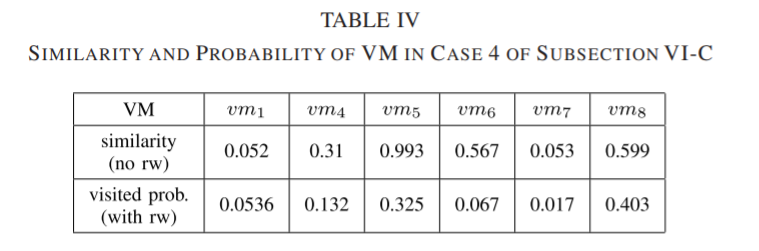# 进程级别的根本原因分析

Storm 应用程序被建模为一个称为拓扑（topology）的有向图，通常包括两种类型的组件：spout 和 bolt ，spout 是数据流的一个源，而 bolt 使用来自 spout 或其他 bolt 的元组，并按照用户代码定义的方式进行处理。Spout 和 bolt 可以在多个虚拟机上并行执行多个任务，即在一个集群中的工作节点中。